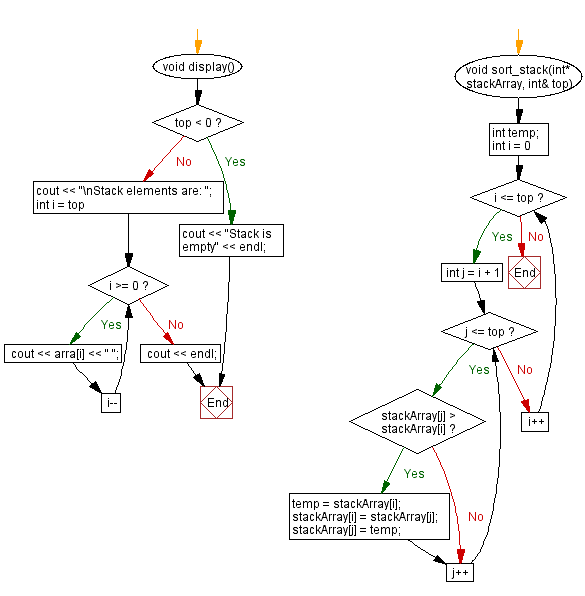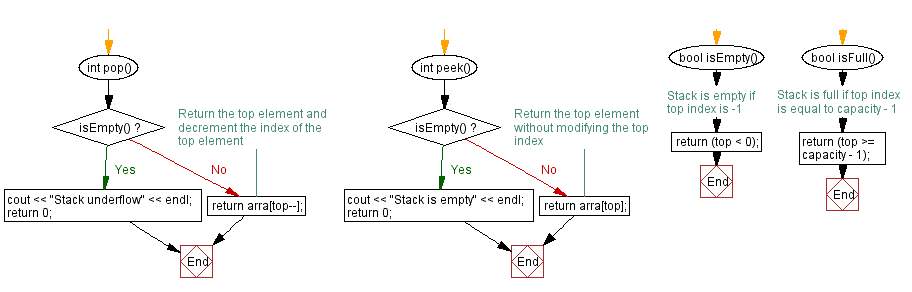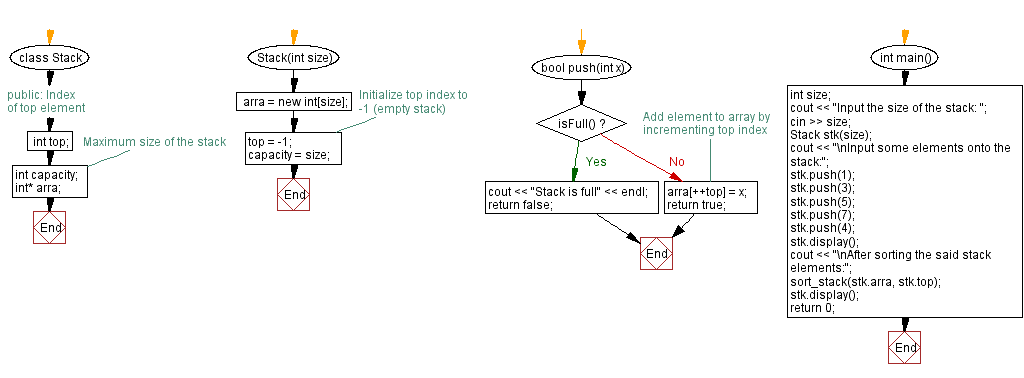﻿ C++ Sort a stack (using a dynamic array) elements

# C++ Stack Exercises: Sort a stack (using a dynamic array) elements

## C++ Stack: Exercise-21 with Solution

Write a C++ program that sorts the stack (using a dynamic array) elements.

Test Data:
Input the size of the stack: 5
Input some elements onto the stack:
Stack elements are: 4 7 5 3 1
After sorting the said stack elements:
Stack elements are: 1 3 4 5 7

Sample Solution:

C++ Code:

``````#include <iostream>

using namespace std;
class Stack {
public:
int top;        // Index of top element
int capacity;   // Maximum size of the stack
int* arra;
Stack(int size) {
arra = new int[size];
top = -1;       // Initialize top index to -1 (empty stack)
capacity = size;
}

bool push(int x) {
if (isFull()) {
cout << "Stack is full" << endl;
return false;
}
//Add element to array by incrementing top index
arra[++top] = x;
return true;
}

int pop() {
if (isEmpty()) {
cout << "Stack underflow" << endl;
return 0;
}
//Return the top element and decrement the index of the top element
return arra[top--];
}

int peek() {
if (isEmpty()) {
cout << "Stack is empty" << endl;
return 0;
}
// Return the top element without modifying the top index
return arra[top];
}

bool isEmpty() {
// Stack is empty if top index is -1
return (top < 0);
}

bool isFull() {
// Stack is full if top index is equal to capacity - 1
return (top >= capacity - 1);
}
void display() {
if (top < 0) {
cout << "Stack is empty" << endl;
return;
}
cout << "\nStack elements are: ";
for (int i = top; i >= 0; i--)
cout << arra[i] << " ";
cout << endl;
}
};
void sort_stack(int* stackArray, int& top) {
int temp;
for (int i = 0; i <= top; i++) {
for (int j = i + 1; j <= top; j++) {
if (stackArray[j] > stackArray[i]) {
temp = stackArray[i];
stackArray[i] = stackArray[j];
stackArray[j] = temp;
}
}
}
}
int main() {
int size;
cout << "Input the size of the stack: ";
cin >> size;
Stack stk(size);
cout << "\nInput some elements onto the stack:";
stk.push(1);
stk.push(3);
stk.push(5);
stk.push(7);
stk.push(4);
stk.display();
cout << "\nAfter sorting the said stack elements:";
sort_stack(stk.arra, stk.top);
stk.display();
return 0;
}
``````

Sample Output:

```Input the size of the stack: 5

Input some elements onto the stack:
Stack elements are: 4 7 5 3 1

After sorting the said stack elements:
Stack elements are: 1 3 4 5 7
```

Flowchart:CPP Code Editor:

Previous C++ Exercise: Sort the elements of a stack (using a linked list).
Next C++ Exercise: Reverse a stack (using a dynamic array) elements.

What is the difficulty level of this exercise?

﻿

## C++ Programming: Tips of the Day

Can you remove elements from a std::list while iterating through it?

You have to increment the iterator first (with i++) and then remove the previous element (e.g., by using the returned value from i++). You can change the code to a while loop like so:

```std::list<item*>::iteratori = items.begin();
while (i != items.end())
{
boolisActive = (*i)->update();
if (!isActive)
{
items.erase(i++);  // alternatively, i = items.erase(i);
}
else
{
other_code_involving(*i);
++i;
}
}
```

Ref : https://bit.ly/3smQY4u